# Ruin probability and infinite time

A couple of weeks ago, I had a discussion with a practitioner, working in some financial company, about ruin, and infinite time. And it reminded me a weird result. Well, not a weird result, but a result I found disturbing, at first, when I was a student (that I rediscovered with the eyes of someone dealing with computational issues, seeing here a difficult theoretical question). Consider a simple ruin problem. A player has wealth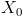. Then he flips a coin: tails he has a gain of 1, heads he experiences a loss of 1. At time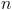, his wealth is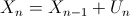where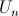is associated to theth coin:is equal to 1 with probability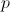(tails), and -1 with probability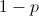(heads). It is also possible to write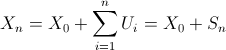where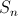can be interpreted as the net gain of the player. In order to get a good understanding of results that can be obtained. Assumeto be given. Let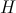denote the number of heads and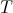the number of tails. Then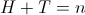, while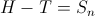. Let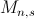denote the number of paths to go from point A (wealth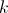at time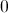) to point B (wealth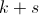at time). Note that this is a Markovian problem, that can be modeled using Markov chainsBut here, we will focus on combinatorial results. Hence,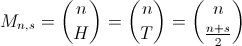In order to derive probabilities to reach, let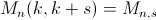denote the number of paths going from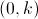to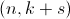. And let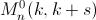denote the number of paths going fromtothat do reachat some point betweenand. Using a simple reflexion property, then ifandare positive,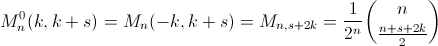Based on those reflexions, two results can be derived (focusing on probability, instead of counting paths). First, we can obtain that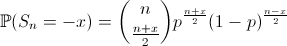(given that n and x have the same parity). The second result we can obtain is that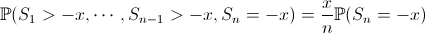Based on those two expressions, if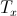denotes the first time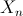become null, given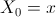,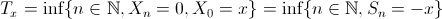then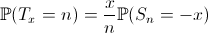This can be computed easily,

```> x=10
> p=.55
> ProbN=function(n){
+ pb=0
+ if(abs(n-x) %% 2 == 0)
+ pb=x/n*choose(n,(n+x)/2)*(1-p)^((n+x)/2)*(p)^((n-x)/2)
+ return(pb)}
> plot(Vectorize(ProbN)(1:1000),type="s")```That looks nice… But if we look closer, we can wonder what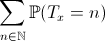would be ? Since we have the distribution of a probabilty measure, we might expect one. But here

```> sum(Vectorize(ProbN)(1:1000))
 0.134385```And this is not due to calculation mistakes that we do not get 1 here. Actually, we should write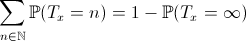which might be interpreted as the probability of ruin, starting from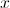, that we denote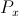from now on. The term on the left can be approximated using monte-carlo simulations

```> p=.55
> x=10
> m=1000
> simul=10000
> S=sample(c(-1,1),size=m*simul,replace=TRUE,prob=c(1-p,p))
> MS=matrix(S,simul,m)
> for(k in 2:m) MS[,k]=MS[,k]+MS[,k-1]
> T0=function(vm) which(vm<=(-x))
> MTmin=apply(MS,1,T0)
> mean(is.na(MTmin)==FALSE)
 0.1328```

To check the validity of the relationship above, a simple (theoretical) recursive formula can be derived for the term on the right (ruin probability), namely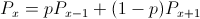with a boundary conditions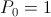, and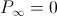. Then is comes that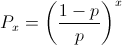Note that it might be tricky to check using monte carlo simulation… since we cannot have an infinite number of runs. And we’re dealing precisely with things that do occur when time is infinite. Actually, we can still check convergence, considering an upper limit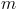for the number of runs, and then lettinggo to infinity. Note that an explicit formula can then be derived (using additional border condition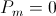)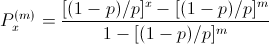Using the following code, it is possible to calculate ruin probability, in order to estimate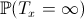.

```> MSmin=apply(MS,1,min)
> mean(MSmin<=(-x))
 0.1328
> (((1-p)/p)^x-((1-p)/p)^m)/(1-((1-p)/p)^m)
 0.1344306```

The following graph shows the evolution of ruin probability as a function of initial wealth (with monte carlo simulation, with a fixed horizon – including a confidence interval – versus the analytical expression)Hence, with stopping times, one should remember that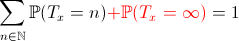and that those two terms can be approximated simply using simulations or standard approximations.